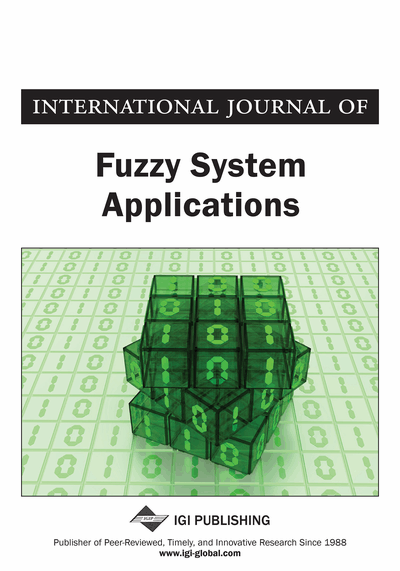# Robust Stabilization And Control Of Takagi-Sugeno Fuzzy Systems With Parameter Uncertainties And Disturbances Via State Feedback And Output Feedback

Iqbal Ahammed A.K., Mohammed Fazle Azeem
DOI: 10.4018/IJFSA.2020070104
OnDemand:
(Individual Articles)
Available
\$37.50
No Current Special Offers

## Abstract

Most of the systems in the industry contain extreme non-linearity and uncertainties, which are hard to design and control utilizing general nonlinear systems. To conquer this sort of troubles, different plans have been produced in the most recent two decades, among which a popular methodology is Takagi-Sugeno fuzzy control. In this article, we present robust stabilization and control of Takagi-Sugeno (T-S) fuzzy systems with parameter uncertainties and disturbances. Initially, Takagi and Sugeno (TS) fuzzy model is used to represent a nonlinear system. Based on this T-S fuzzy model, fuzzy controller design schemes for state feedback and output feedback is also developed. Then, necessary conditions are derived for robust stabilization in the intelligence of Lyapunov asymptotic stability and are expressed in the arrangement of linear matrix inequalities (LMIs). The proposed system is implemented in the working platform of MATLAB and the simulation results are provided to illustrate the effectiveness of the proposed methods.
Article Preview
Top

## 1. Introduction

In practice, most plants are nonlinear and comprise uncertainties. During the past decades, several people have dedicated a lot of effort to both theoretical research and implementation methods to handle nonlinear control problems. Based on this, various control schemes have been developed, one of the most important of which is fuzzy logic theory. Since then this field has been explored further, both theoretically and practically, among which a popular usage method is fuzzy control (Chen, (2011)). In conventional modeling a single system is modeled to define the entire characteristics of the global non-linear system. A fuzzy controller or model uses fuzzy rules, which are linguistic if-then statements involving fuzzy sets, fuzzy logic, and fuzzy inference (Pereira, Nunes de Almeida et al., 2015). Fuzzy rules play a key role in representing expert control knowledge and experience in linking the input variables of fuzzy controllers to output variable. Two major types of fuzzy rules exist, namely, Mamdani fuzzy rules (Davood & Khooban, 2015) and Takagi-Sugeno (TS) fuzzy rules. The constructions of a fuzzy model represent an important and basic procedure in this approach. In general, there are two approaches for constructing fuzzy models, fuzzy modeling using input-output data and derivation from given nonlinear system equations (Morente-Molinera, Perez, et al., 2015).

To design a T-S fuzzy controller, we need a T-S fuzzy model for a nonlinear system. TS fuzzy model is widely accepted as a powerful modeling tool for describing complex nonlinear plants, which has been successfully applied into industrial control. T-S fuzzy model define the non-linear system into simple sub-linear modules and define the global characteristics of the nonlinear system. (Tao, Taur et al., 2010). TS fuzzy system since it is a powerful solution that bridges the gap between linear and nonlinear control systems (Qiu, Feng et al., 2010). The important advantage of the T–S fuzzy system is its universal approximation of any smooth nonlinear function by a “blending” of some local linear system models, which greatly facilitates the analysis and synthesis of the complex nonlinear system (Jiang, Gao et al., 2011; Nian and Zheng, 2012). The complex non-linear plant can be described as a weighted sum of linear state equations (Lai, Wu et al., 2015; Xie, Yue et al., 2016). In order to meet control objectives, conceptually, there exist two distinct approaches to design a fuzzy adaptive control system: direct and indirect schemes. In the direct method, a fuzzy system is used to describe the control action and the parameters of the fuzzy system are adjusted directly to meet the control objective (Dong & Wang, 2009).

Unlike the direct schemes, the indirect adaptive approach uses fuzzy systems to estimate the plant dynamics and then a control law is designed based on these estimates. In indirect adaptive schemes, the possible controller singularity problem usually meets (Shi, Zhang et al., 2011). Also, various algorithms and schemes are developed for the fuzzy adaptive control system. An observer-based indirect adaptive fuzzy neural tracking controller combined with a variable structure scheme and H∞ control algorithms is developed for nonlinear MIMO systems involving plant uncertainties and external disturbances (Hui Zhang, Yang Shi, et al., (2013)) (Li, Liu et al., 2012). The reliable fuzzy H∞ controller is designed to guarantee the system’s asymptotic Stability. To further overcome the fuzzy approximation error and external disturbances, the adaptive fuzzy controllers are enlarged with a robust compensator, namely a sliding mode controller (SLM), robust adaptive controller or with an H∞ tracking controller (Nekoukar & Erfanian, 2011).

## Complete Article List

Search this Journal:
Reset
Volume 13: 1 Issue (2024)
Volume 12: 1 Issue (2023)
Volume 11: 4 Issues (2022)
Volume 10: 4 Issues (2021)
Volume 9: 4 Issues (2020)
Volume 8: 4 Issues (2019)
Volume 7: 4 Issues (2018)
Volume 6: 4 Issues (2017)
Volume 5: 4 Issues (2016)
Volume 4: 4 Issues (2015)
Volume 3: 4 Issues (2013)
Volume 2: 4 Issues (2012)
Volume 1: 4 Issues (2011)
View Complete Journal Contents Listing